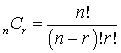A combination is a listing in which the order of listing does not matter. This describes the number of ways that we can choose r objects from a total of n objects, where the order in which the r objects is listed does not matter (The combination formula, or the binomial formula):If you want to change selection, open document below and click on "Move attachment"

Subject 10. Principles of Counting
nlike the multiplication rule, factorial involves only a single group. It involves arranging items within a group, and the order of the arrangement does matter. The arrangement of ABCDE is different from the arrangement of ACBDE. <span>A combination is a listing in which the order of listing does not matter. This describes the number of ways that we can choose r objects from a total of n objects, where the order in which the r objects is listed does not matter (The combination formula, or the binomial formula): For example, if you select two of the ten stocks you are analyzing, how many ways can you select the stocks? 10! / [(10 - 2)! x 2!] = 45. &